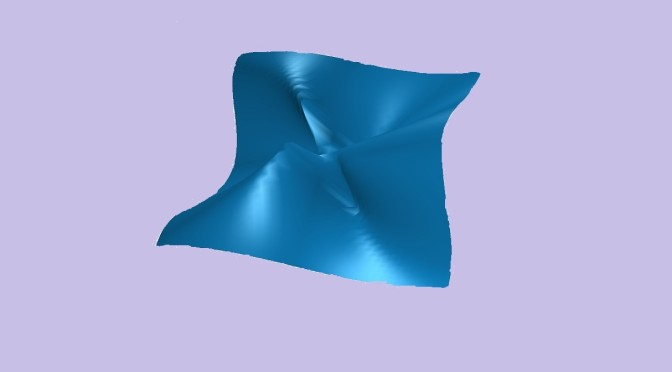Continuity of multivariable real functions

This article provides counterexamples about continuity of functions of several real variables. In addition the article discusses the cases of functions of two real variables (defined on $$\mathbb R^2$$ having real values. $$\mathbb R^2$$ and $$\mathbb R$$ are equipped with their respective Euclidean norms denoted by $$\Vert \cdot \Vert$$ and $$\vert \cdot \vert$$, i.e. the absolute value for $$\mathbb R$$.

We recall that a function $$f$$ defined from $$\mathbb R^2$$ to $$\mathbb R$$ is continuous at $$(x_0,y_0) \in \mathbb R^2$$ if for any $$\epsilon > 0$$, there exists $$\delta > 0$$, such that $$\Vert (x,y) -(x_0,y_0) \Vert < \delta \Rightarrow \vert f(x,y) - f(x_0,y_0) \vert < \epsilon$$.

A discontinuous function at the origin whose partial functions are continuous

Consider the function $\begin{array}{l|rcl} f : & \mathbb R^2 & \longrightarrow & \mathbb R \\ & (x,y) & \longmapsto & \frac{xy}{x^2+y^2} \text{ for } (x,y) \neq (0,0)\\ & (0,0) & \longmapsto & 0 \end{array}$ For $$x \in \mathbb R$$ we have $$f(x,0)=0$$ hence the function $$x \mapsto f(x,0)$$ is continuous. Similarly, the function $$y \mapsto f(0,y)$$ is continuous as it is also always vanishing.

However, $$f$$ is not continuous at the origin $$(0,0)$$ as for $$x \in \mathbb R \setminus \{0\}$$ we have $$f(x,x)=\frac{x x}{x^2 + x^2}=\frac{1}{2}$$ while $$f(0,0)=0$$. Apart on the $$x$$-axis and $$y$$-axis, $$f$$ is discontinuous on the other lines passing through $$(0,0)$$. In fact for $$\lambda \neq 0$$, we have $f(x,\lambda x) = \frac{x \lambda x}{x^2 + (\lambda x)^2}=\frac{\lambda}{1+\lambda^2} \neq 0$ while $$f(0,0) = 0$$.

A function discontinuous at the origin, but continuous on all lines passing through the origin

Next we look at $\begin{array}{l|rcl} g : & \mathbb R^2 & \longrightarrow & \mathbb R \\ & (x,y) & \longmapsto & \frac{x^2 y}{x^4+y^2} \text{ for } (x,y) \neq (0,0)\\ & (0,0) & \longmapsto & 0 \end{array}$ The functions $$x \mapsto g(x,0)$$ and $$y \mapsto g(0,y)$$ are both constant and equal to zero, and are therefore continuous. And for $$\lambda \neq 0$$ and $$x \neq 0$$: $g(x,\lambda x) = \frac{x^2 \lambda x}{x^4 + (\lambda x)^2}=\frac{\lambda x}{x^2+\lambda^2}$ hence $\lim\limits_{x \to 0, x \neq 0} g(x, \lambda x) = g(0,0)=0$ which proves that $$g$$ is continuous at $$(0,0)$$ on all lines passing through the origin. However for $$x \neq 0$$, $$g(x,x^2)=\frac{1}{2}$$. Consequently, $$g$$ is not continuous at $$(0,0)$$ as the curve $$x \mapsto (x,x^2)$$ is passing through $$(0,0)$$.

A two variables function continuous only at $$(0,0)$$

The function $\begin{array}{l|rcl} h : & \mathbb R^2 & \longrightarrow & \mathbb R \\ & (x,y) & \longmapsto & \Vert (x,y) \Vert \text{ for } (x,y) \in \mathbb Q^2\\ & (x,y) & \longmapsto & 0 \text{ otherwise}\end{array}$ is continuous at $$(0,0)$$ as for $$(x,y) \in \mathbb R^2$$, $$\Vert h(x,y) \Vert \le \Vert (x,y) \Vert$$.

However $$h$$ is discontinuous at $$(x,y) \neq (0,0)$$. For the proof, we notice that $$\mathbb Q$$ is dense in $$\mathbb R$$. So one can find a sequence $$((x_n,y_n))_{n \in \mathbb N}$$ of $$\mathbb Q^2$$ with $$(x_n,y_n) \to (x,y)$$ hence $$\lim\limits_{n \to \infty} h(x_n,y_n) = \Vert (x,y) \Vert >0$$. While $$\mathbb R \setminus \mathbb Q$$ is also dense in $$\mathbb R$$ so for a sequence $$((x_n,y_n))_{n \in \mathbb N}$$ of $$(\mathbb R \setminus \mathbb Q)^2$$ with $$(x_n,y_n) \to (x,y)$$ we have $$\lim\limits_{n \to \infty} h(x_n,y_n) = 0$$.